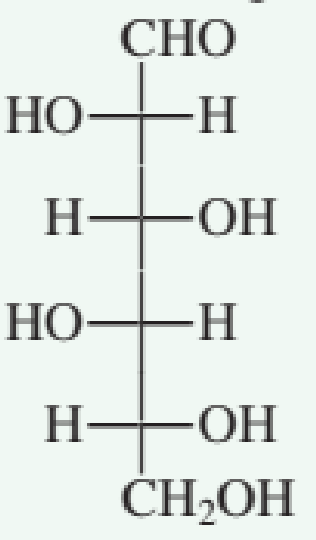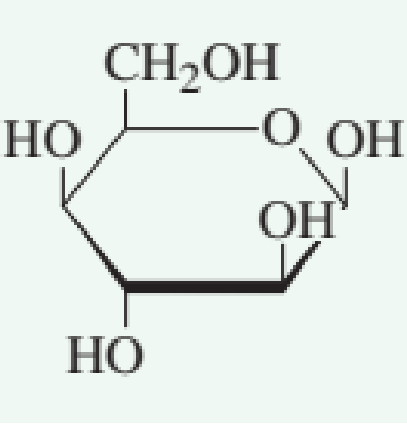Chapter 7.11, Problem 2QQ### Organic And Biological Chemistry

7th Edition
STOKER + 1 other
ISBN: 9781305081079

#### Solutions

Chapter
Section### Organic And Biological Chemistry

7th Edition
STOKER + 1 other
ISBN: 9781305081079
Textbook Problem

# Which of the following is the correct Haworth projection formula for a monosaccharide with the Fischer projection formulaa. no correct response

Interpretation Introduction

Interpretation: The correct Haworth projection formula for a monosaccharide with the given Fisher projection formula has to be predicted.

Concept introduction: The structural representation of sugar molecule in cyclic form is known as Haworth projection.  The substituents which are present on the right side in the Fischer projection are drawn below the ring in the Haworth projection.  Similarly, the substituents which are present on the left side in the Fischer projection are drawn above the ring in the Haworth projection.

Explanation

Reason for correct option:

The given Fisher projection formula of a monosaccharide is shown below.

The CH2OH group is attached to the first carbon to the left of the oxygen atom of ring.  It is drawn up because the monosaccharide is D-aldohexose.  An OH group is added to the anomeric carbon and each of the remaining three carbon atoms

### Still sussing out bartleby?

Check out a sample textbook solution.

See a sample solution

#### The Solution to Your Study Problems

Bartleby provides explanations to thousands of textbook problems written by our experts, many with advanced degrees!

Get Started

#### Find more solutions based on key concepts